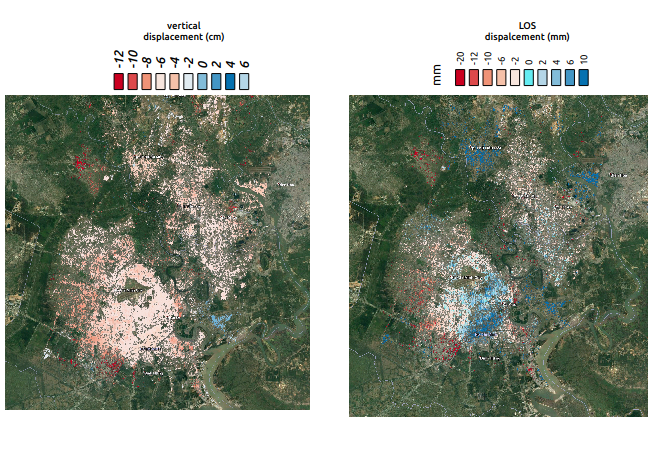Difference between vertical and LOS displacement

Hello everyone,
I am trying to estimate building subsidence for the area of Ho Chi Minh in Vietnam. I have used 6 interferograms (between June 2016 and June 2017) where I stacked them and produced the subsidence map.

Firstly, I produced displacement in LOS geometry using the following equation. unwrapped (phase * wavelength) / (-4 * PI) (or ‘phase to displacement’ from SNAP where the results are the same). I also calculated the vertical displacement using the following equation: (unwrapped phase * wavelength) / (-4 * PI * rad(cos(incidence angle))). The results are shown below.We can see, on the felt, we have the vertical displacement in cm and on the right the LOS displacement in mm. We notice that there is a big difference in values between vertical and LOS displacement. The results of vertical displacement look better (we do not have uplift in the main city, just subsidence, as expected) while in LOS, we see that the vertical displacement values ( from the image on the left) became positive which indicates an uplift (blue values).

I do not understand why the results look different between LOS and vertical displacement. I expected to get the same results in both vertical and LOS geometry.

4 Likes

Did you use incidence-angle over the ellipsoid?

1 Like

hi mengdahl,

For calculating the vertical displacement, I used the local incidence angle.

The finding of two Similar studies for the same area were a LOS displacement between 5 and 10mm. In my case, it shows 4 to 6 mm uplift. While the results with the vertical displacement are much better.

I do not know where the problem lies. It is very strange

1 Like

Hi, Johngan,

I am new in this field, and will do the same thing as you did ( find the displacement from the unwrapped phase ). I think the equation you used is correct, yet do not know the detailed operation steps ( after opening the product with the un-wrapped phase band, click which menu item, fill what parameters, … ). Can you tell me any tutorial materials available? Thank you!

Hi liniC,

ESA has released three nice books of interferometry. It starts from basics and it gets more advanced. Please , see the link below

Also, a good tutorial for ground subsidence is given in the following link. https://saredu.dlr.de/unit/insar_deformation

1 Like

besides johngan’s recommendation, maybe this topic helps you as well:

You need to use the local incidence angle over the ellipsoid (which is varying very slowly) and not the one derived from the DEM (that is varying according to the local topography).

1 Like

I have not though about that. I will use the incidence angle over the ellipsoid

I would be interested in the results and how this makes a difference.

sure, I will post the results and make a comparison between the results derived using local incidence angle and the results derived using the incidence angle over the ellipsoid.

Hi again @mengdahl,

I just want to correct myself. For deriving the vertical subsidence, I have used indeed the incidence angle from ellipsoid (and not the local incident angle as I first mentioned. Sorry for the confusion) as shown below which varies from 32.4 to 34.4 deg. While the local incidence angle varies from 19 to 42 deg.

I guess it would not make much sense to use the local incidence angle for deriving the vertical displacement, as it varies a lot as you mentioned.

@johngan Using the formula for calculating the displacement in LOS you provided in your first post I obtained strange results. Using unwrapped phase in the range -37 to 18 the calculated displacement is quite different compared to the displacement obtained with the SNAP function . Any idea?

Thank you.

Hi Hriston+bg,

I imagine you are referring to the following equation: (phase * wavelength) / (-4 * PI)
When you use SNAP tool for deriving LOS displacement, the units are in meters. Hence, if you want to get exactly the same numbers in LOS with that of SNAP, when using the equation make sure that the units in wavelength are in meters. If you enter the units in cm, the results will be in cm.

Using either the equation or the SNAP toolbox the results should be the same

@johngan Could you please put the number you set? I used wavelength=5.6 for cm as units.

please, take a look at this post. Phase to Displacement Units?

The values for the equation are the following:
(unwrapped phase * 0.056) / (-4 * PI).
the results will give you LOS displacement in meters just like SNAP

@johngan Thank you!! I’ll try it.

1 Like

Hi all,

Sorry but, I am confused just the issue of wavelenght, How can you see the value of wavelenght or can you calculate this value?

Best regards
Fikret

Wavelength depends on the sensor, you can find it with Google. You can also look up the radar frequency from the abstracted metadata and compute wavelength = speed of light/frequency.

Hi @mengdahl,

I am using Sentinel 1B IW SLC data. Does anyone know the wavelenght of this data?

Fikret